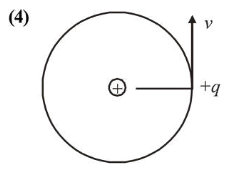# A charged particle going around in a circle can be considered to be a current loop.

Question:

A charged particle going around in a circle can be considered to be a current loop. A particle of mass $m$ carrying charge $q$ is moving in a plane wit speed $v$ under the influence of magnetic field $\vec{B}$. The magnetic moment of this moving particle :

1. (1) $\frac{m v^{2} \vec{B}}{2 B^{2}}$

2. (2) $-\frac{m v^{2} \vec{B}}{2 \pi B^{2}}$

3. (3) $-\frac{m v^{2} \vec{B}}{B^{2}}$

4. (4) $-\frac{m v^{2} \vec{B}}{2 B^{2}}$

Correct Option: , 4

Solution:Length of the circular path, $l=2 \pi r$

Current, $i=\frac{q}{T}=\frac{q v}{2 \pi r}$

Magnetic moment $M=$ Current $\times$ Area

$=i \times \pi r^{2}=\frac{q v}{2 \pi r} \times \pi r^{2}$

$M=\frac{1}{2} q \cdot v \cdot r$

Radius of circular path in magnetic field, $r=\frac{m v}{q B}$

$\therefore M=\frac{1}{2} q v \times \frac{m v}{q B} \Rightarrow M=\frac{m v^{2}}{2 B}$

Direction of $\vec{M}$ is opposite of $\vec{B}$ therefore

$\vec{M}=\frac{-m v^{2} \vec{B}}{2 B^{2}}$

(By multiplying both numerator and denominator by $B$ ).